Some basic wireless concepts:Free space path loss formula:

Forexample, at L-band, let's say 1 GHz, we have

Pr - Pt (LOSS) = Gr+Gt -32.44-60-20log x(km)

Assuming antenna gains of 0 dB, at 10km we have.

Loss = -112.44 dB

This means our transmitted signal should be 112.44 dBm to receive 0dBm signal.
Every 10 km will result in 6dB more loss.

so we can conclude two times distance ~ 6 dB loss

Two times Frequency ~ 0.5 Range

Two times frequency ~ 6 dB loss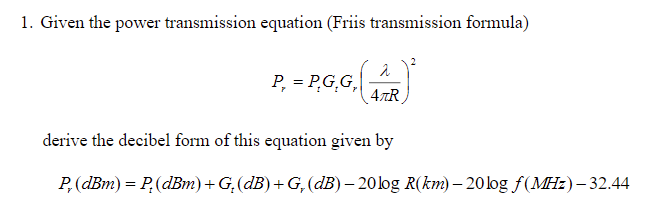Phase noise:

Phase noise is the noise spectrum that is seen spreading out either side of a signal as a result of the phase jitter that exists. Noise arising from the short term phase fluctuations that occur in a signal. The fluctuations manifest themselves as sidebands which appear as a noise spectrum spreading out either side of the signal.B

Phase noise is of particular importance to RF designer.

Express in dBc/Hz. which means: dB down in level from the carrier proportional to the bandwidth.

e.g. when we say phase noise is -100dBc/Hz @ 100 Hz : This means when frequency offset is 100 Hz, the phase noise is -100dBc/Hz

Noise Type Frequency Dependence Phase Slope/Decade
White phase f0 0
Flicker phase f−1 −10
White frequency f−2 −20
Flicker frequency f−3 −30
Random walk f−4 −40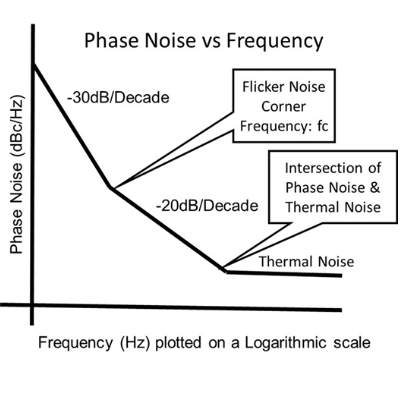What is IP3:

In amplifiers, we have input power and output power. when we say this amplifier have 10dB gain forexample, we never mention the range of input power. Most amplifiers have linear relation between input power and output power but this ends at some point. this linear perios is called "linear response" after this linear region is "Compression region". Forexample we have 1 dB compression point where we see increase of output power 1 dB but we had to increase input power forexample 1.4 dB.
Now IP3 or intercept point, is when we increase input power but output power will be constant.Do you have extra band to use? why not do spread spectrum?

Advantage: difficult to detect, interfere and jam!

Types: Direct Sequence Spread Spectrum (DSSS)-->each bit convert to multiple bits

Frequency Hopping Spread Spectrum (FHSS)--> each bit broadcast in random series of frequencies

Chirp Spread Spectrum (CSS)--> each bit swift in frequency with different start and end

OFDM:

*Orthogonal Frequency Division Multiplexing

*Why? Higher data rate How? multi carrier  --> resilient to interference

*Disadvantage: 1. High PAPR (Peak to average power ratio) 2. Sensitive to frequency offset and drift.

*Examples: WiFi, LDACS, FBMC, 4G and 5G.

*The receiver has a bank of demodulators, bring each carrier to baseband.

Difference FDM vs OFDM --> Orthogonality of subbands carrier, FDM needs guard band

ISI problem: Delayed version of a symbol overlaps with the adjacent symbol. Solution: give a guard band. but wait! receiver can't transmit zero! solution Cyclic prefix (CP): copy and paste part of symbol from tail to its front.MIMO: Multiple Input Multiple Output
Types:
1. Time diversity

2. Frequency diversity

3. Spatial diversity

4GLTE, 5G.

-----

VSWR or Voltage Standing Wave Ratio = 1+Reflection_coefficient/1-Reflection_coefficent

Return Loss: How much power is reflected and how much absorbed =20logZ_L - Z_0  /  Z_L + Z_0
where Z is impedance and Z_L is impedance at load and Z_0 is initial impedance
Z_0 = sqrt(L/C)

a measure of impedance matching of loads to the characteristic impedance of a transmission line or waveguide. Impedance mismatches result in standing waves along the transmission line.

Return Loss = 20log (VSWR+1) / (VSWR-1)

Isolator: allows signal to flow in one way and prevent reflection to damage previous component. Usually, we use it at the output of the amplifier

What is an antenna figure of merit? Gain / Antenna noise temperature (how much external noise collected by receiving antenna)

RF circulator: two RF isolators in one direction! so three-port circulator pass signal from port 1 to 2, from 2 to 3, and from 3 to 1. not reverse!

Effective Isotropic Radiated Power (EIRP): Power that transmitted! TX power+antenna gain

Noise Figure (NF) or Noise Factor(F):

You may heared the noise figure of this device is blaa blaa! what does that mean? Noise figures show how the signal to noise ratio (SNR) is degraded by that device! or the amount of noise power added by electronic parts in the receiver to the thermal noise power fron the input of the receiver.

F = SNR_input - SNR_output

NF= 10*log(F)

Cascading Noise figure in a system:

Assume four devices in our receiver design with noise factor of F1, F2, F3 and F4:

The overall noise factor F is = F1+ F2-1/G1 + F3-1/(G1G2) + F4 - 1/(G1G2G3)

MixerRF Analog Impairments:

* Amplifier nonlinearities (described above)

* Oscillator phase noise / Jitter

* Carrier Frequency Offset (CFO)/Jitter or Sampling Frequency Offset (SFO)

* I/Q mismatch

Sensitivity:

Simple definition: The weakest signal that a receiver can identify and process! see figure.Formula: Sensitivity=10log(Thermal_Noise) +NF + SNR

where Thermal_Noise is kTB --> k (Boltzmann's constant) X T(room tempreture) X B (bandwidth)

------Forexample-------------- 1.38 x10^-23    X  290    X  1 Hz  =-203 dBW/Hz or -173.9 dBm/Hz

------Forexample-------------- 1.38 x10^-23    X  290    X  1 MHz  = -114 dBm/HzForexample many commercial GPS receiver sensitivity is around -167 dBm.

P_sensitivity = -174 + 10logBW + SNR + NF

Phase Lock Loop (PLL):

Simple definition: A circuit or chip that detects the phase of the input signal and generates the same signal with it's VCO.The Voltage Controlled Oscillator, VCO, within the PLL produces a signal which enters the phase detector. Here the phase of the signals from the VCO and the incoming reference signal are compared and a resulting difference or error voltage is produced. This corresponds to the phase difference between the two signals.

The error signal from the phase detector passes through a low pass filter which governs many of the properties of the loop and removes any high frequency elements on the signal. Once through the filter the error signal is applied to the control terminal of the VCO as its tuning voltage. The sense of any change in this voltage is such that it tries to reduce the phase difference and hence the frequency between the two signals. Initially the loop will be out of lock, and the error voltage will pull the frequency of the VCO towards that of the reference, until it cannot reduce the error any further and the loop is locked.

When the PLL, phase locked loop, is in lock a steady state error voltage is produced. By using an amplifier between the phase detector and the VCO, the actual error between the signals can be reduced to very small levels. However some voltage must always be present at the control terminal of the VCO as this is what puts onto the correct frequency.

The fact that a steady error voltage is present means that the phase difference between the reference signal and the VCO is not changing. As the phase between these two signals is not changing means that the two signals are on exactly the same frequency. [A1]

Phase detector example:Many RF and wireless standards dictate a spectrum mask for the industrial communication devices that their emission should satisfy a mask!
This mask indicates the spurious and phase noise levels for transmission.

For example:GPS, GLONASS:

GPS:Figure: GPS transmitter [B1]

GPS vs GLONASS: GPS-->CDMA || GLONASS--> FDMA

Table I: [B2]

GLONASS GPS
Constellation Number of satellite 24 32
Number of orbits 3 6
Orbital inclination 64.8° 55°
Orbital radius 25,510 km 26,560 km
Orbital altitude 19,130 km 20,200 km
Orbit period 11 h 15.8 min 11 h 58 min
Signal characteristics Multiplexing FDMA CDMA
Carrier frequencies 1602 + k × 0.5625 MHz
1246 + k × 0.4375 MHz
1575.42 MHz
1227.60 MHz
Code frequencies C/A code: 0.511
P code: 5.11
C/A code: 1.023
P code: 10.23
Broadcast ephemerides Position, velocity, acceleration Keplerian elements
Coordinates system PZ-90.02 WGS-84
Time system GLONASS time GPS time

Smith Chart:

Using this chart you can find the impedance of a transmission line and antenna system as a function of frequency. These days people use ADS software.

RTL (Register Transfer Level) vs SLD (Sequential Logic Design):

In RTL Design the basic building blocks are registers, Multiplexers, Adders. In Sequential Logic Design the basic building blocks are the Logic Gates, Flip-Flops. RTL is more data flow approach but SLD is more hardware in nature.Coherent Time:

Electromagnetic: the time over which a propagating wave may be considered coherent, meaning that its phase is, on average, predictable.

Communication: the time duration over which the channel impulse response is considered to be not varying (e.g. Doppler)

More explanation: the time domain dual of Doppler spread and is used to characterize the time varying nature of the frequency dispersiveness of the channel in the time domain. The Maximum Doppler spread and coherence time are inversely proportional to one another. T_c = 1/f_m

where f_m is the maximum Doppler spread or maximum Doppler shift

Coherent Bandwith:

A bandwidth (range of freq) which channel can be considered flat. Similarly to coherent time, it has inversely proportional to delay spread.

B_c = 1/D. where D is the delay spread in second.

if the coherent bandwidth (B_c) > Signal Bandwidth ==> channel is flat.

Rake receiver, which exploits multipath delay components to improve the performance of the system. A rake receiver combines the information from several correlators, each one tuned to a different path delay, producing a stronger version of the signal than a simple receiver with a single correlation tuned to the path delay of the strongest signal.

In other words,

a radio receiver designed to counter the effects of multipath fading. It does this by using several "sub-receivers" called fingers, that is, several correlators each assigned to a different multipath component. Each finger independently decodes a single multipath component; at a later stage the contribution of all fingers are combined in order to make the most use of the different transmission characteristics of each transmission path. This could very well result in higher signal-to-noise ratio (or Eb/N0) in a multipath environment than in a "clean" environment.

The idea to boost the strong signal components and attenuate the weak (relatively noisy) components, as performed in MRC diversity, is exactly the same as the type of filtering and signal weighting used in the matched filter receiver. A particularly interesting application of this concept is the Rake receiver for detecting direct-sequence CDMA signals over a dispersive channel.

The rake receiver consists of multiple correlators, in which the receive signal is multiplied by time-shifted versions of a locally generated code sequence. The intention is to separate signals such that each finger only sees signals coming in over a single (resolvable) path. The spreading code is chosen to have a very small autocorrelation value for any nonzero time offset. This avoids crosstalk between fingers.In practice, the situation is less ideal. It is not the full periodic autocorrelation that determines the crosstalk between signals in different fingers, but rather two partial correlations, with contributions from two consecutive bits or symbols. It has been attempted to find sequences that have satisfactory partial correlation values, but the crosstalk due to partial (non-periodic) correlations remains substantially more difficult to reduce than the effects of periodic correlations. The rake receiver is designed to optimally detected a DS-CDMA signal transmitted over a dispersive multipath channel. It is an extension of the concept of the matched filter.

Central Limit Theorem:

The central limit theorem states that if you have a population with mean μ and standard deviation σ and take sufficiently large random samples from the population with replacement text annotation indicator, then the distribution of the sample means will be approximately normally distributed. This will hold true regardless of whether the source population is normal or skewed,

Shanon Theorem:

Tightest upper bound on the information rate of data (C) that can be communicated at an arbitrarily low error rate using an average received signal power through an analog communication channel subject to additive white Gaussian noise (AWGN) of power:

C = bandwidth X (1 + SNR)

Stationary Process  wide sense stationary(WSS):

A random process that parameters such as mean and variance do not change over time. e.g. white noise.

The wide sense stationary uncorrelated scattering (WSSUS) model is commonly used for multipath fading channels. WSSUS channel assumes that the channel correlation function is invariant over time, and that the scatterers with different path delays are uncorrelated. The WSSUS assumption is realistic to describe the short-term variations of the radio channel.

Wiener–Khintchine theorem:

States that the autocorrelation function of a wide-sense-stationary random process has a spectral decomposition given by the power spectrum of that process or simply

PSD is equal to the Fourier transform of the autocorrelation function(treated as a function of the delay).

why this theorem important? in LTI system. The Fourier transform of the autocorrelation function of the output of an LTI system is equal to the product of the Fourier transform of the autocorrelation function of the input of the system times the squared magnitude of the Fourier transform of the system impulse response

GFSK VS FSK:

Converts the digital baseband signals (0 or 1) to passband frequency modulated signals.

FSK modulated signals have better noise immunity in comparison with the amplitude modulated signals, and FSK modulated systems are less complicated for demodulation.

Gaussian (GFSK) modulation is the Gaussian filter applied FSK modulation. GFSK uses a
Gaussian filter to smooth the beginning of each digital symbol before the frequency modulation
procedure.Antenna Diversity:

2 types: SIMO and MIMO (single/multiple inputs multiple outputs)

Why?

Because: *Diversity Gain *Fading mitigation *Security

maximum-ratio combining (MRC):
The idea is to boost the strong signal components and attenuate the weak (relatively noisy) components, as performed in MRC diversity,

e.g. in BPSK, Expressing in decibels, with two receive antennas, we GAIN 3dB.

for N RX antenna: BER=0.5*erfc(sqrt(N*Eb/N0))

Lease square in maximum-ratio-combining (MRC):which means that the signal from each antenna is rotated and weighted according to the phase and strength of the channel, such that the signals from all antennas are combined to yield the maximum ratio between signal and noise terms.From Channel Impulse Response to Delay/Doppler Power Spectrum :CDMA:IQ Imbalance :

IQ imbalance is a performance-limiting issue in the design of direct conversion receivers, also known as zero intermediate frequency (IF) or homodyne receivers. Such a design translates the received radio frequency (RF, or pass-band) signal directly from the carrier frequency (fc) to baseband using only one mixing stage. The traditional heterodyne receiver structure needs an IF stage between the RF and baseband signals. The direct conversion receiver structure does not have an IF stage and does not need an image rejection filter. Due to the lower component count, it is easier to integrate. However, a direct-conversion RF front-end suffers from two major drawbacks: one is IQ imbalance and the other is DC offset. When designing a homodyne receiver, control of IQ imbalance is necessary to limit signal demodulation error.

IQ imbalances occur due to mismatches between the parallel sections of the receiver chain dealing with the IQ signal paths. The local oscillator (LO) generates a sine wave and a copy of that sine wave that is delayed by 90 DEGREES. When the direct LO output is mixed with the original signal, this produces the I signal, whereas when the delayed LO output is mixed with the original signal, that produces the Q signal. In the analog domain, the delay is never exactly 90 degrees. Similarly, the analogue gain is never perfectly matched for each of the signal paths.

Minimum Mean Square Error (MMSE)

An estimation method in signal processing

We have prior information about the range of a parameter (Bayesian situation)(e.g. noise)

We can make better posterior estimates as more observations become available.

Assume n is a random variable (e.g. noise) and X is input data to the system.

n has a probability density function of p(n)

X and n have joint pdf of p(n,X)

MMSE estimation of n = n^ = g(X) that minimizes E[(n-n^)^2)]

n^ = E[n|X] = integral(n*p(n|X)dn)

Flicker noise or pink noise or 1/f noise:

Definitions:

*the noise power is inversely proportional to the frequency

*often occurs as a resistance fluctuation

*Passive components have 1/f noise and current noise

*low resistances the 1/f noise and current noise are usually too small to be considered

##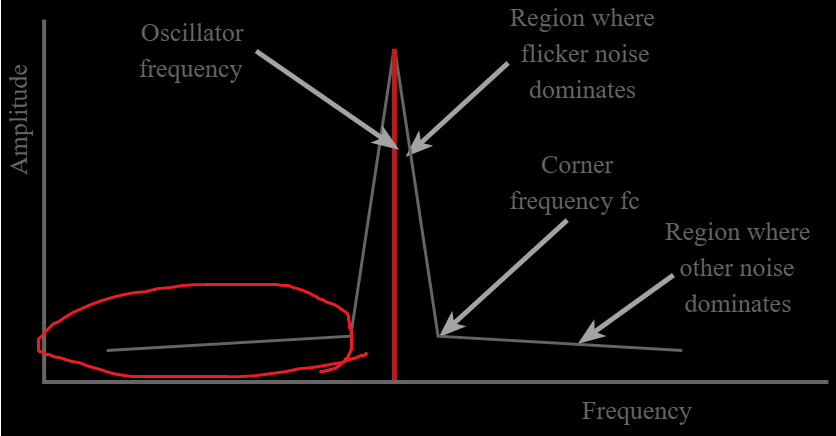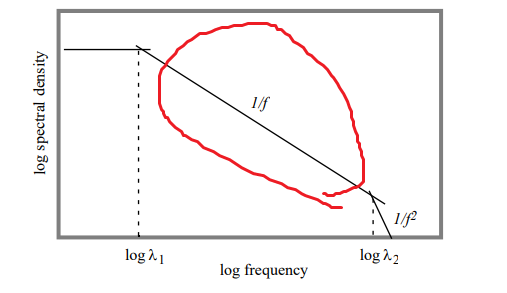How to remove flicker noise?

Answer: Chopper stabilization works by alternating or chopping the input signals at the input stage and then chopping the signals again at the output stage. This is the equivalent to modulation using a square wave. Chopper stabilization, or chopping, is a technique to reduce amplifier offset voltage.

first the low-frequency signal is shifted to high frequency by multiplying it with high-frequency carrier, and it is given to the device affected by the flicker noise. The output of the device is again multiplied with the same carrier, so the previous information signal comes back to baseband, and flicker noise will be shifted to higher frequency, which can easily be filtered out.

Difference between FIR (Finite Implulse Response) and IIR (Infinite Impulse Response) filters?:

 FIR IIR Linear Phase Response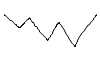Nonlinear Phase Response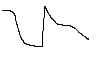Group delay is better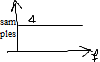Gourp delay is not good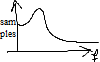More memory Less Memory Only depends on Input Depends on both input and output Only zeros both zeros and poles (numerator and denumerator) Easy to implement Difficunt to implement Tapping high order Tapping lower order

FIR filter: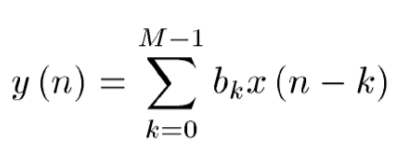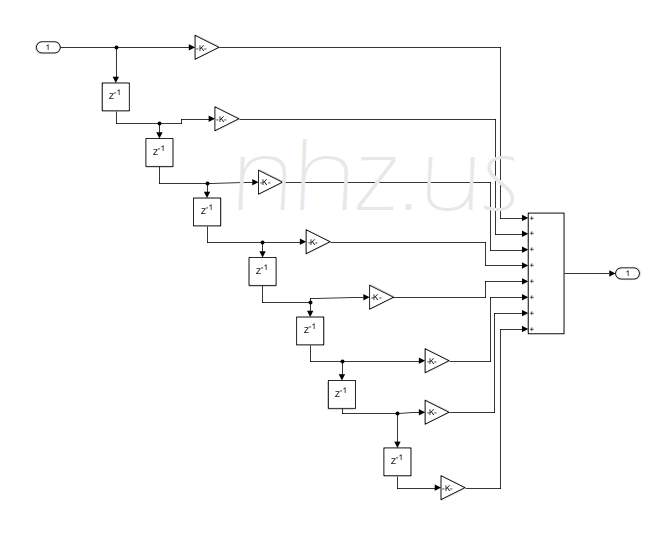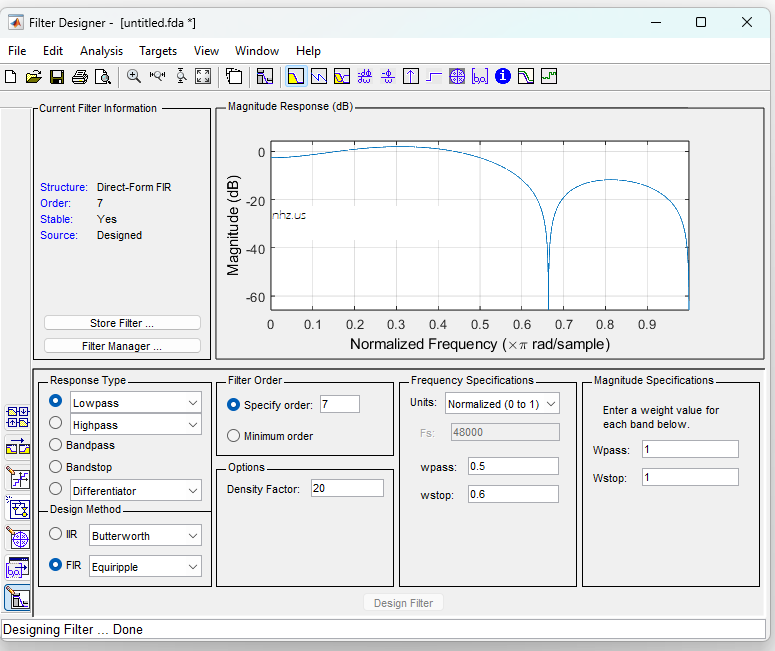1) Open Matlab "filterdesigner" --> Get coefficients --> change filter structure to get nominator and denominator for IIR

3) Replace coefficients

IIR Filter: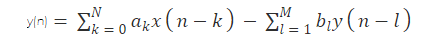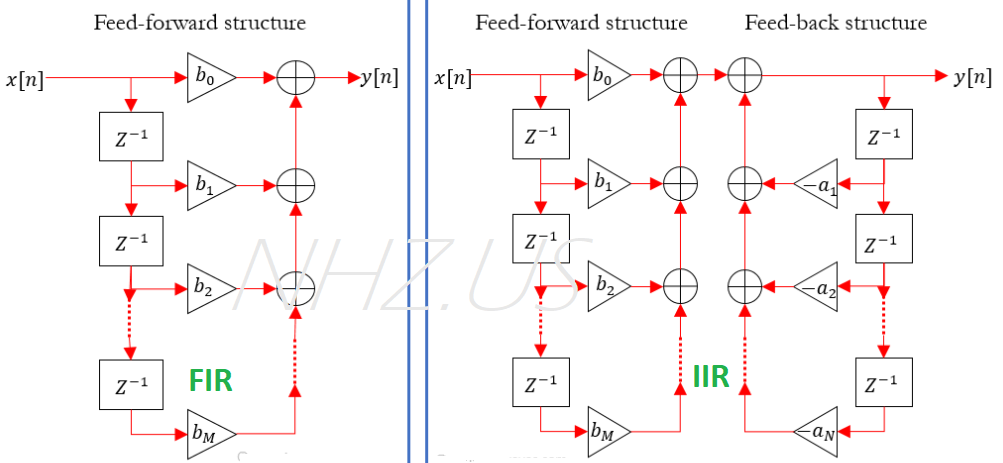Red --> IIR Blue --> FIR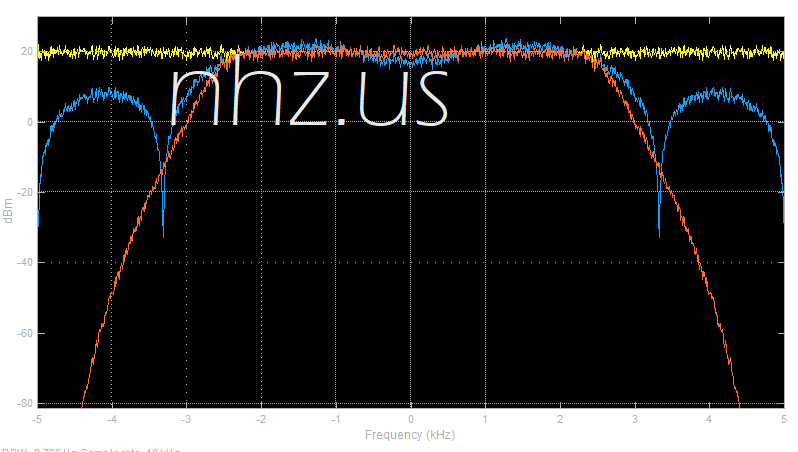MLSE (Maximum Likelihood Sequence Estimation) in simple words

Estimate the most likely sequence of transmitted symbols in a communication system.

In communication systems, the transmitted signals may get distorted due to various factors such as noise, interference, and multipath propagation. MLSE helps to estimate the original transmitted sequence by finding the sequence that is most likely to have produced the received signal, given the knowledge of the channel and the statistical properties of the transmitted symbols.

Two general steps:
1) received signal is compared to all possible transmitted symbols (Sequence Estimation) --> sensitivity vs complexity -->better if there is a relationship between received signal and different symbol periods e.g. convolutional coding, continues phase modulation

2)  calculate the likelihood of each possible sequence of transmitted symbols (Maximum Likelihood) --> value of squared Euclidean distances between hypothetic signals and the received signal --> sometimes simplified to cross correlation

Find Markov model --> state of signal --> intersymbol relationship or correlation e.g. MSK phase changes by 1/2*pi at each sample time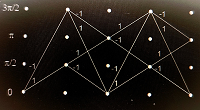or in case of QPSK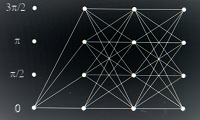which in QPSK there is no dependency from symbol to symbol so no benefit to decode a sequence

Flicker noise or pink noise or 1/f noise:

Local Oscilator Feed Through, LO Leakage in zero-IF architecture